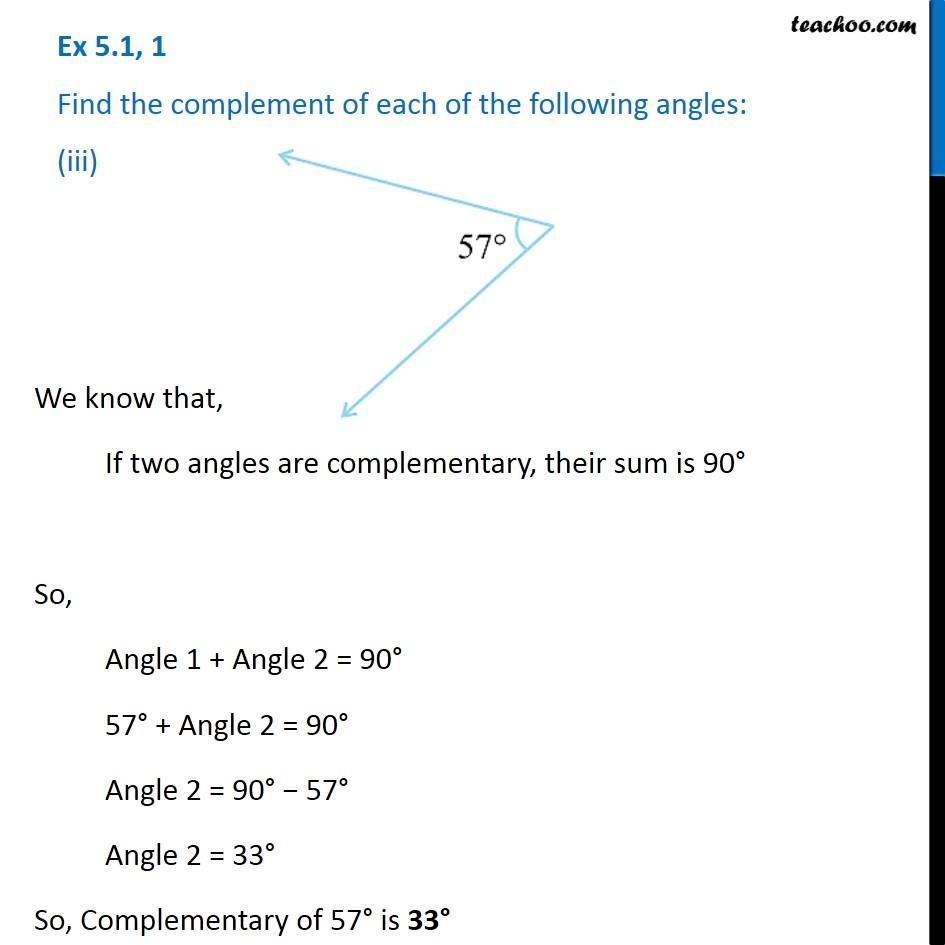Ex 5.1

Chapter 5 Class 7 Lines and Angles
Serial order wiseLearn in your speed, with individual attention - Teachoo Maths 1-on-1 Class

### Transcript

Ex 5.1, 1 Find the complement of each of the following angles: (iii)We know that, If two angles are complementary, their sum is 90° So, Angle 1 + Angle 2 = 90° 57° + Angle 2 = 90° Angle 2 = 90° − 57° Angle 2 = 33° So, Complementary of 57° is 33°